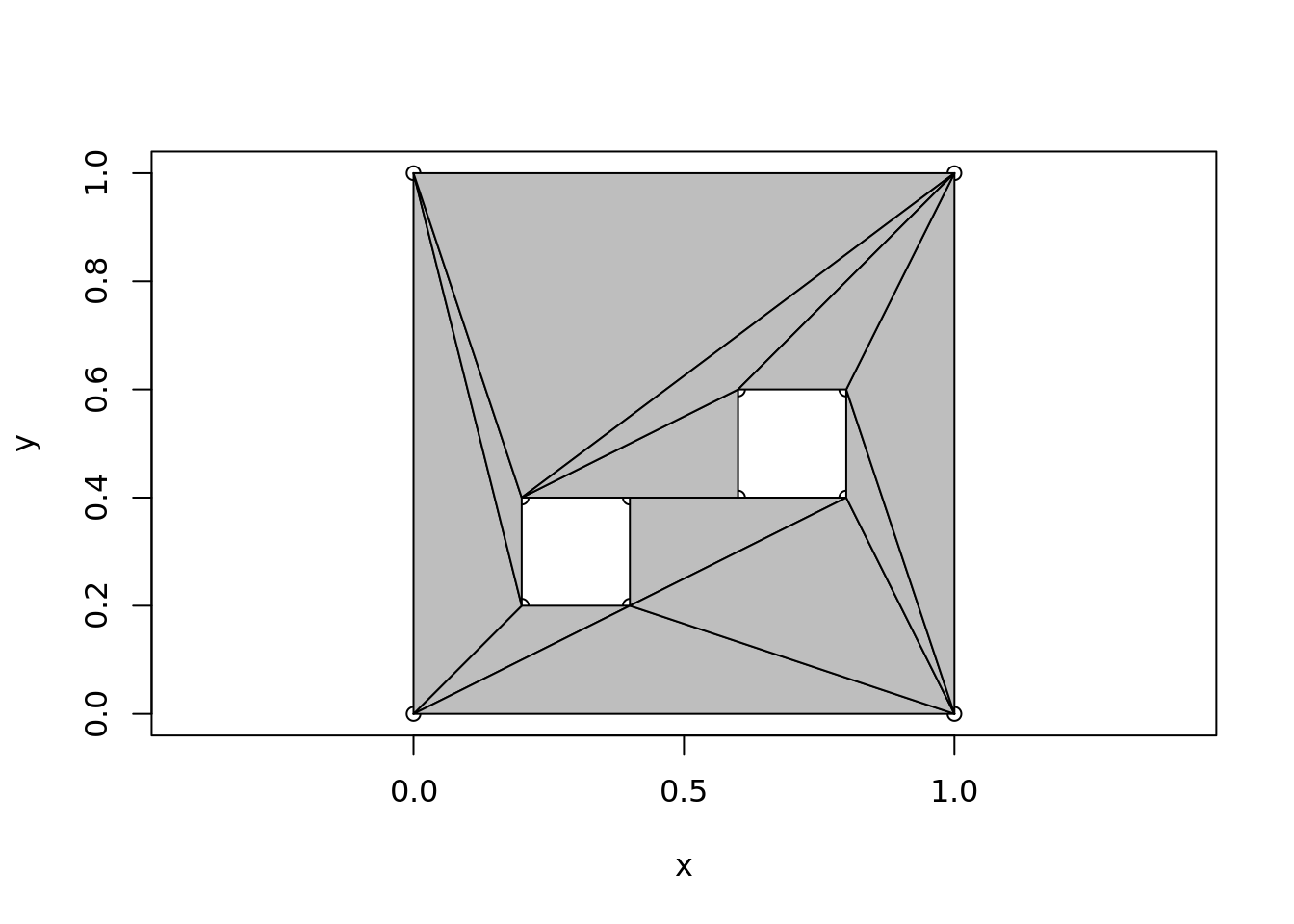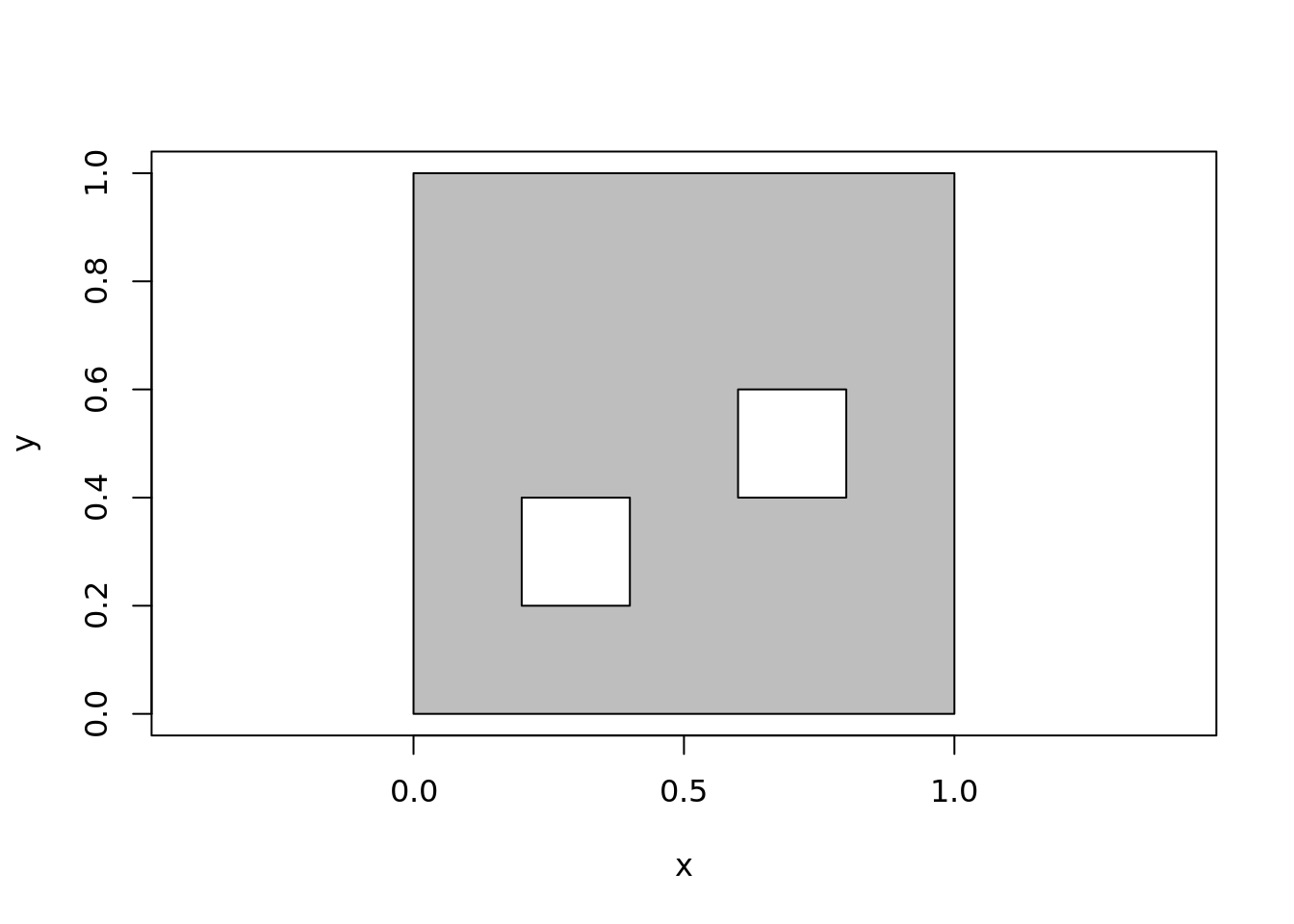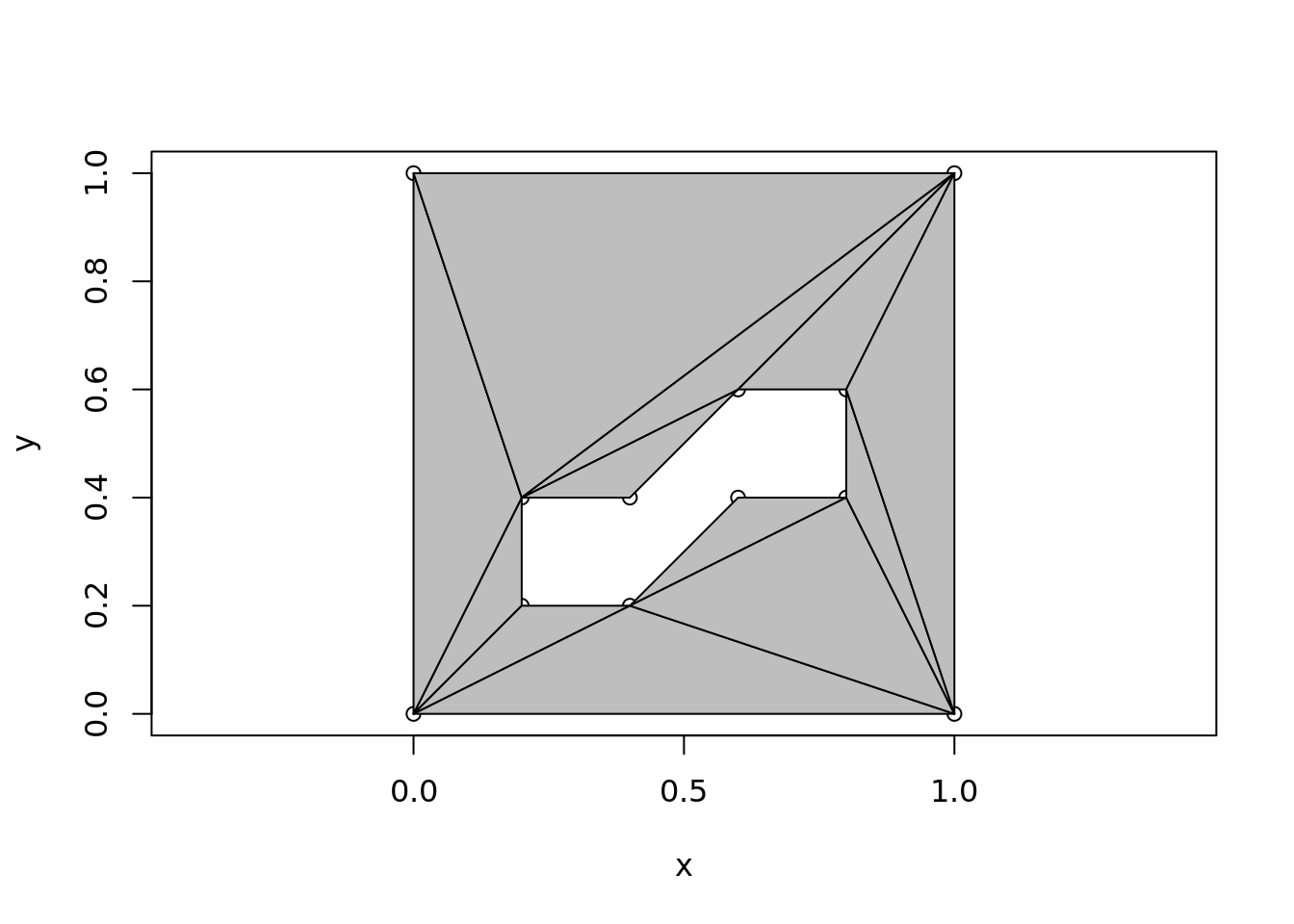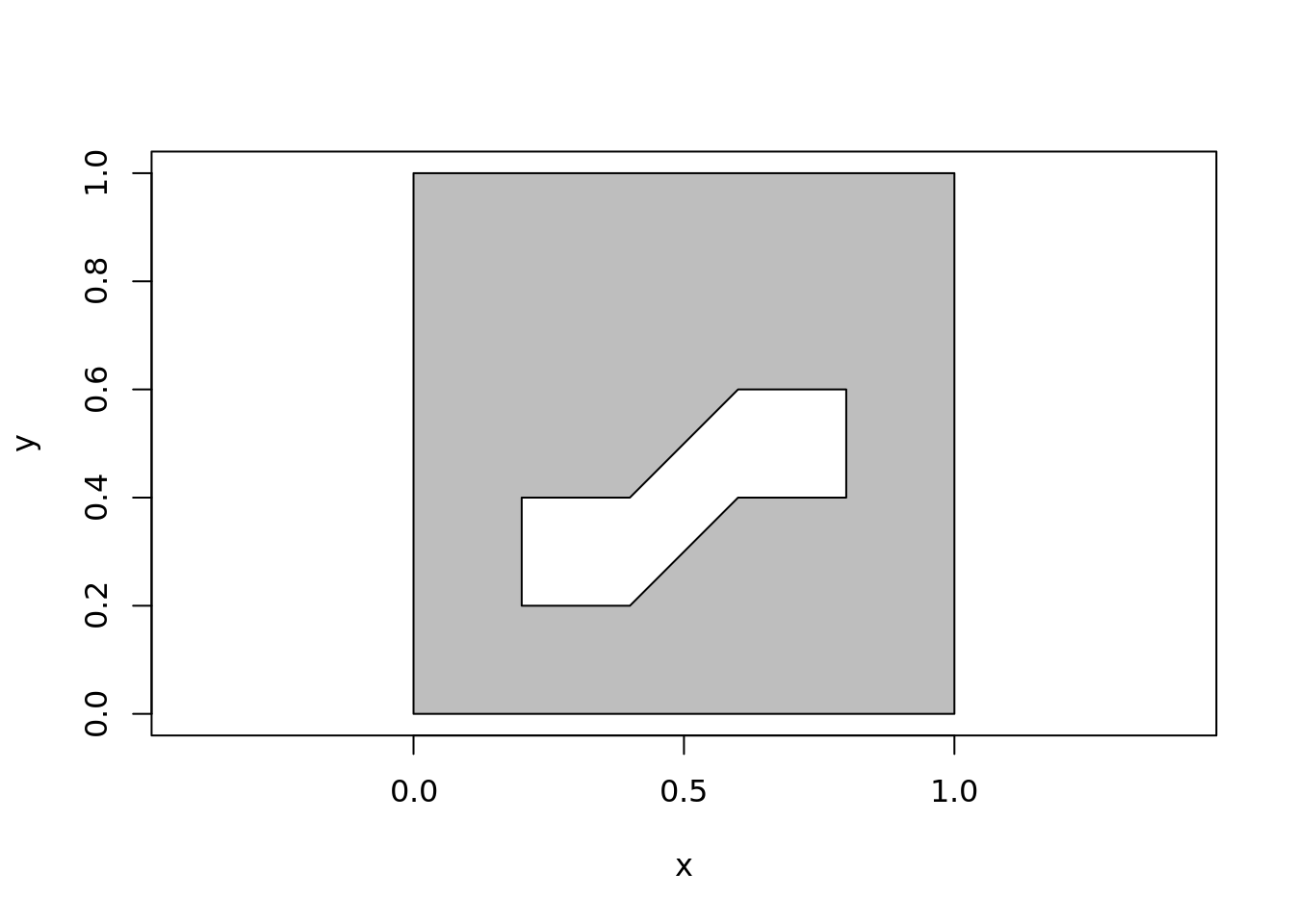## more than one hole
## but the two inside holes are open to each other
## (so we can use the same data for one hole or two)
x <- c(0, 0, 1, 1,
0.4, 0.2, 0.2, 0.4,
0.6, 0.8, 0.8, 0.6
)
y <- c(0, 1, 1, 0,
0.2, 0.2, 0.4, 0.4,
0.6, 0.6, 0.4, 0.4
)
library(decido)
ind <- decido::earcut(x, y, holes = c(5, 9))
plot_ears(cbind(x, y), ind, col = "grey")## NULL
plot_holes(cbind(x, y), holes = c(5, 9), col = "grey")## now run with just the first hole indicated, they merge as one
ind <- decido::earcut(x, y, holes = 5)
plot_ears(cbind(x, y), ind, col = "grey")## NULL
plot_holes(cbind(x, y), holes = 5, col = "grey")## extra check, ensure the JS gives the same result

ind <- rearcut::earcut(cbind(x, y), holes = c(5, 9))
plot_ears(cbind(x, y), ind, col = "grey")## NULL
plot_holes(cbind(x, y), holes = c(5, 9), col = "grey")## now run with just the first hole indicated, they merge as one
ind <- rearcut::earcut(cbind(x, y), holes = 5)
plot_ears(cbind(x, y), ind, col = "grey")## NULL
plot_holes(cbind(x, y), holes = 5, col = "grey")